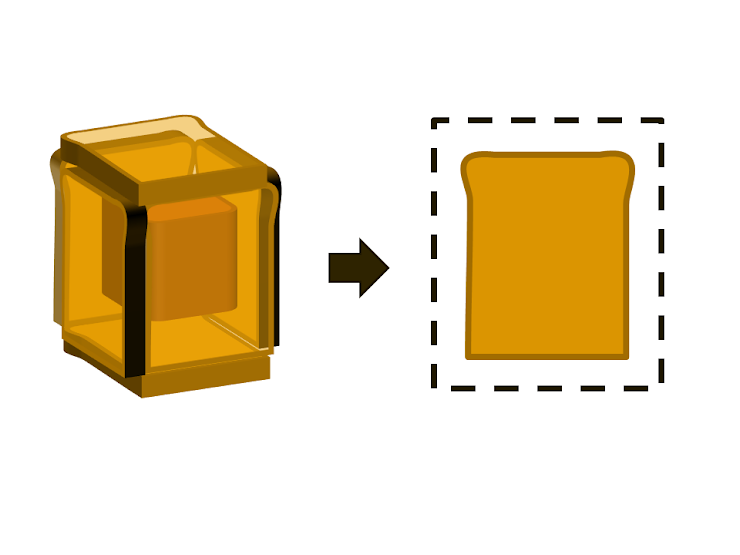Counting Sandwiches
This survey is about ground-breaking research into the effects of division and construction of bread on the frequency of sandwiches.

If you object to them being called sandwiches (e.g. "These are soldiers!"), answer 0.
How many sandwiches are shown in the box? *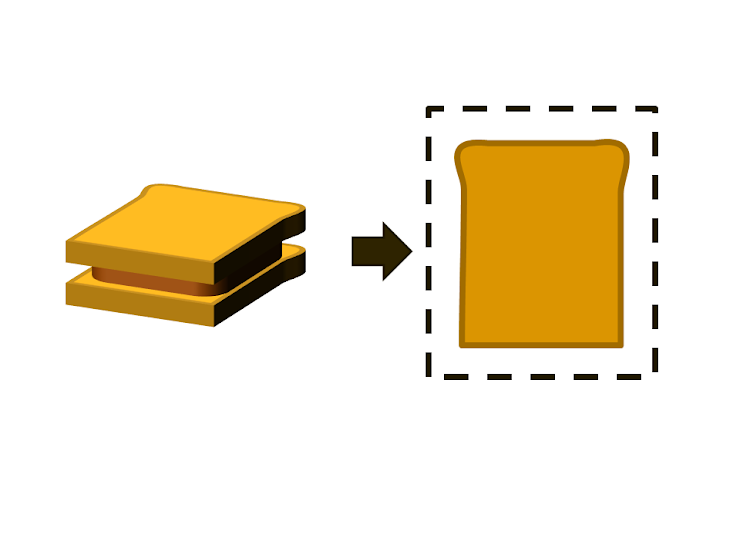How many sandwiches are shown in the box? *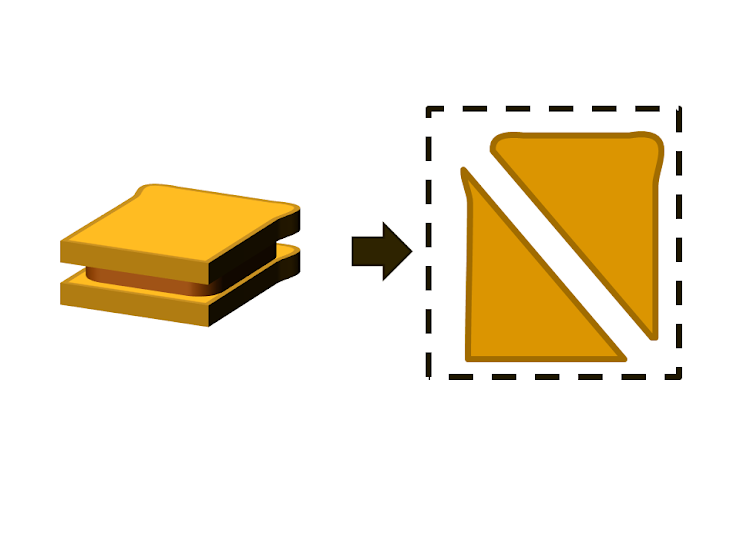How many sandwiches are shown in the box? *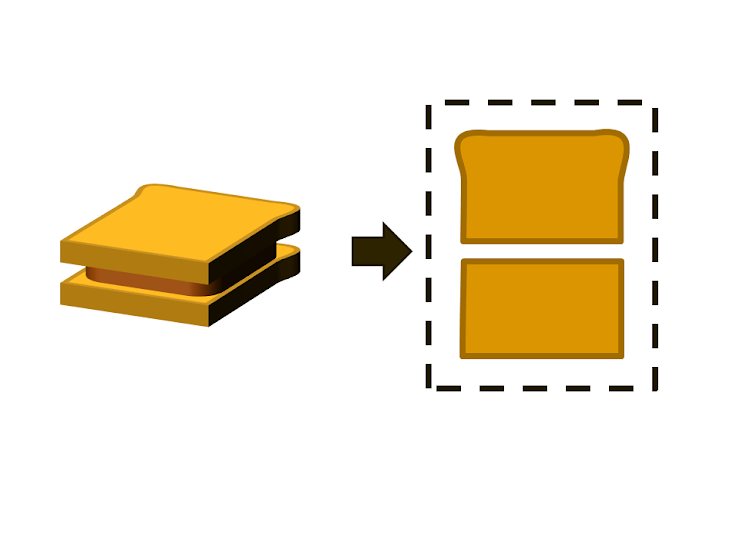How many sandwiches are shown in the box?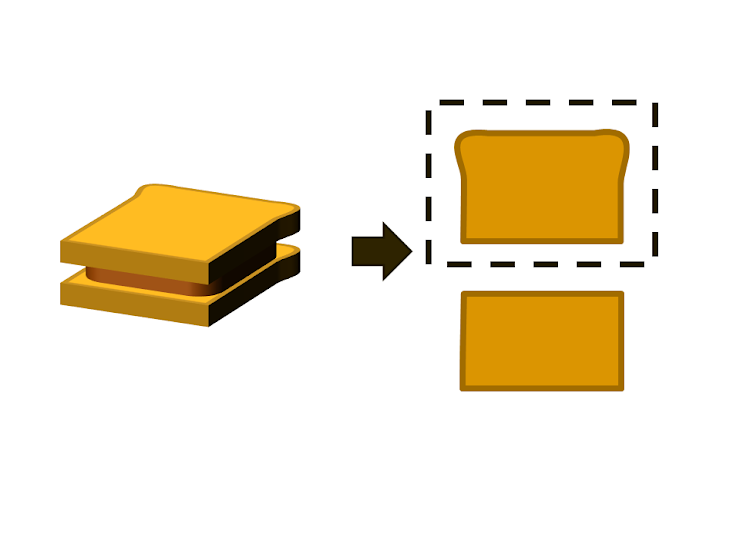How many sandwiches are shown in the box?How many sandwiches are shown in the box?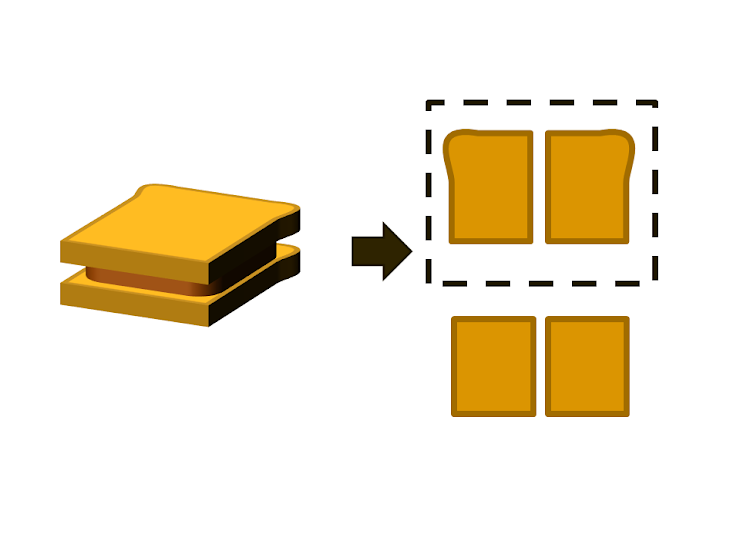How many sandwiches are shown in the box?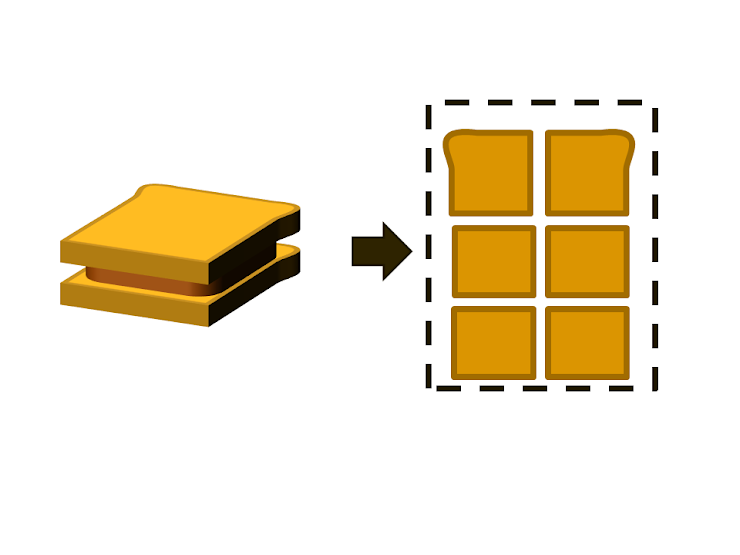How many sandwiches are shown in the box?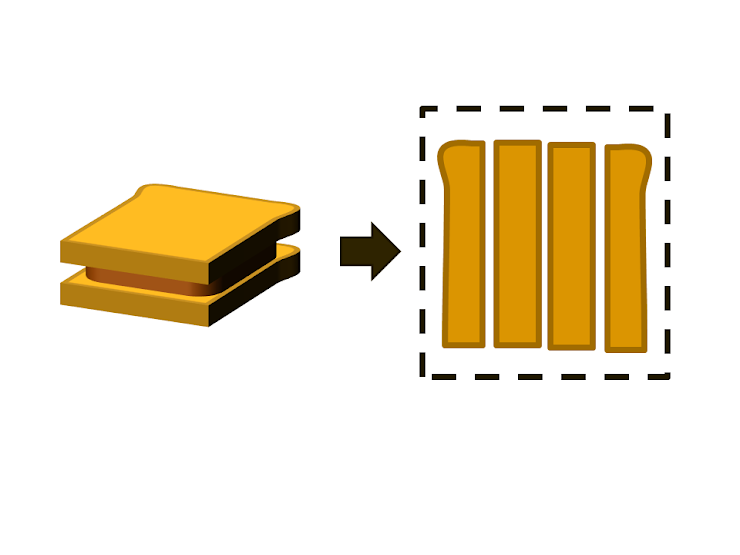How many sandwiches are shown in the box?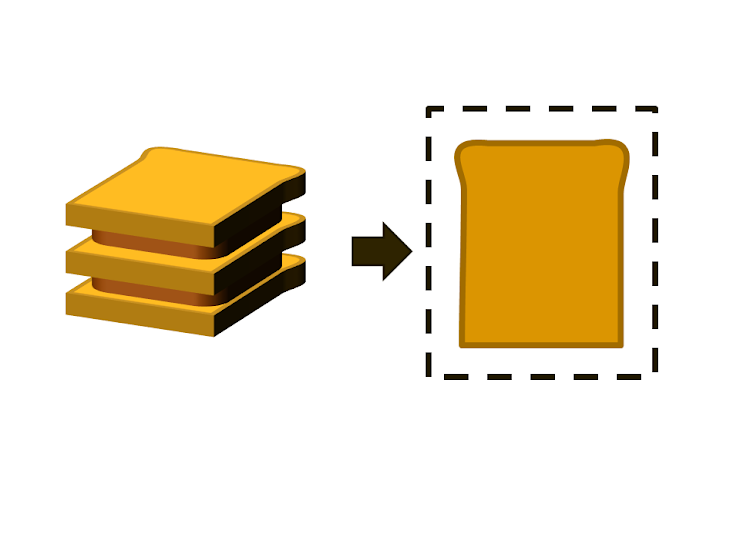How many sandwiches are shown in the box?How many sandwiches are shown in the box?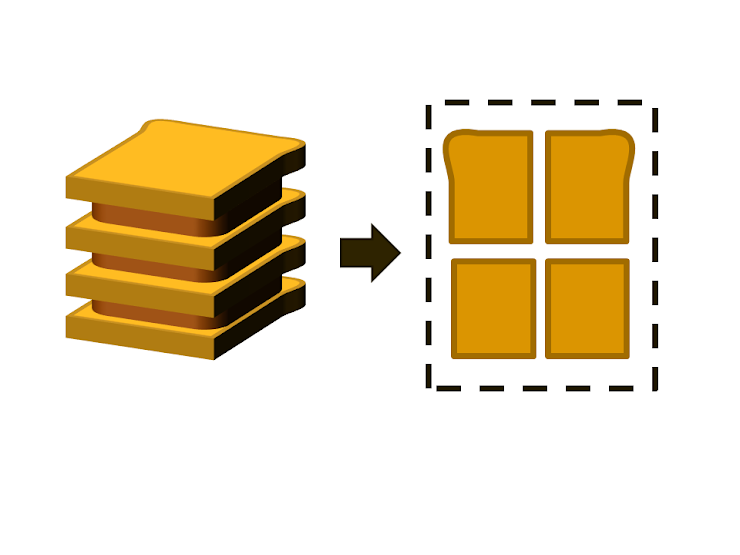How many sandwiches are shown in the box?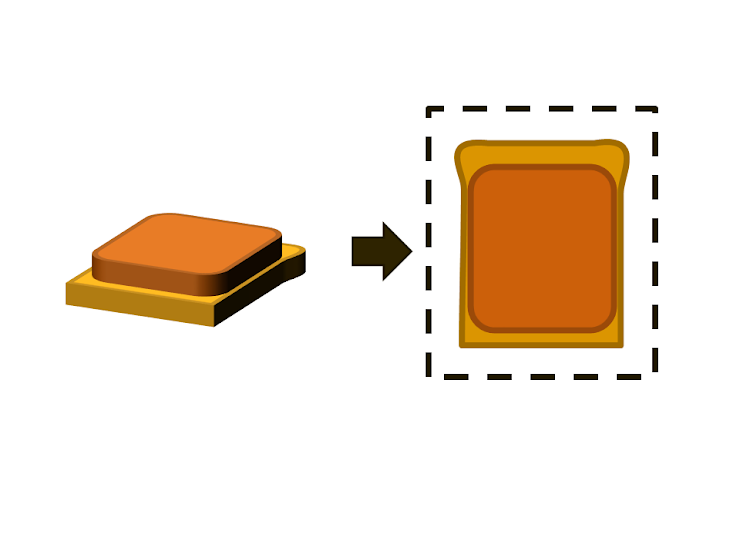How many sandwiches are shown in the box? (this sandwich is folded.)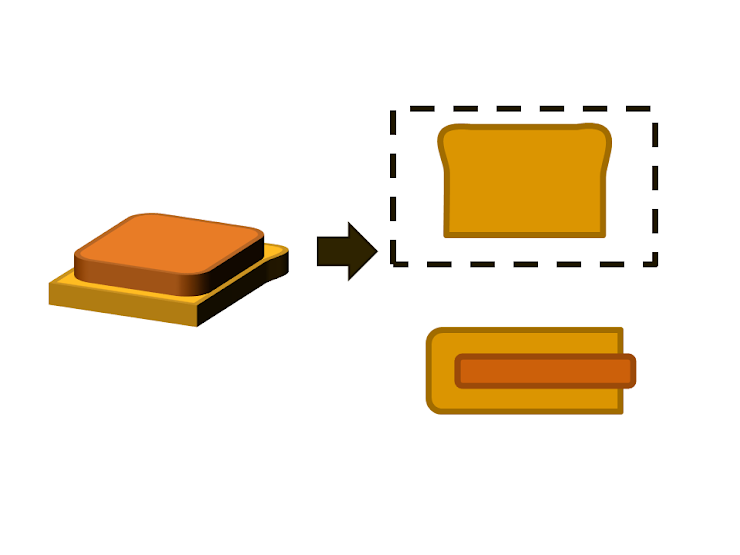How many sandwiches are shown in the box?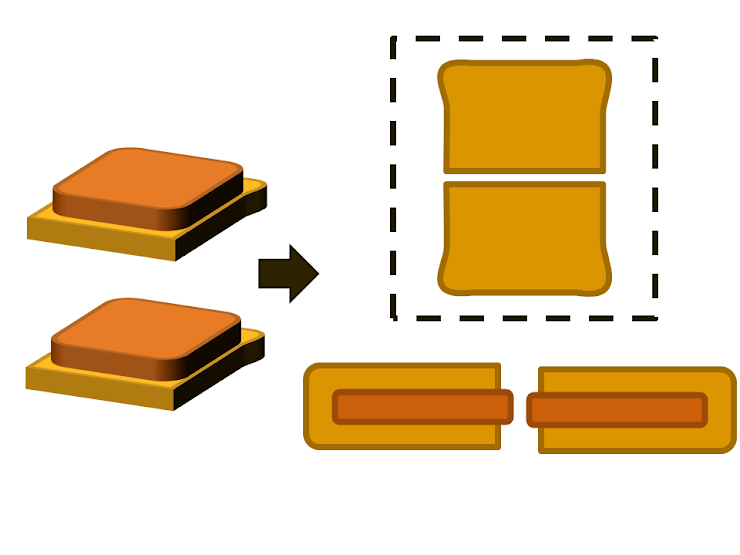How many sandwiches are shown in the box?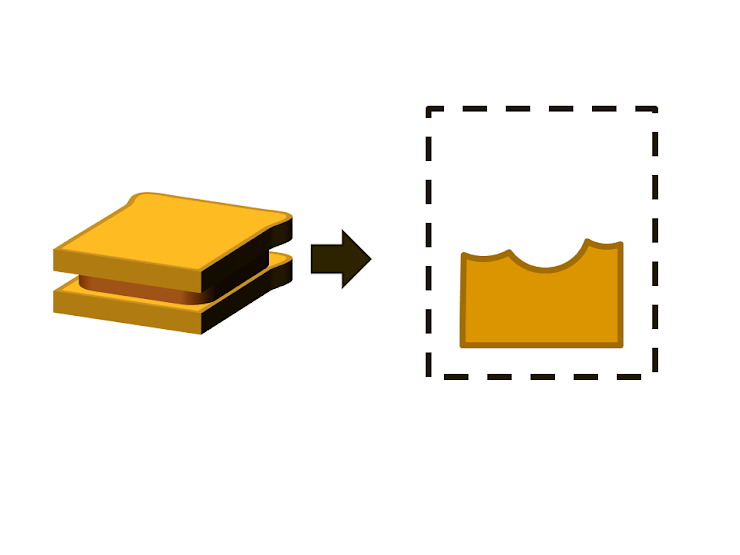How many sandwiches are shown in the box?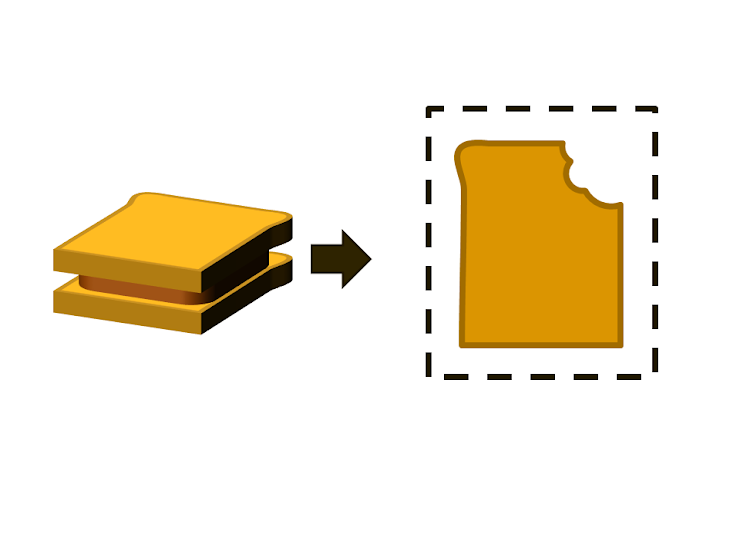How many sandwiches are shown in the box?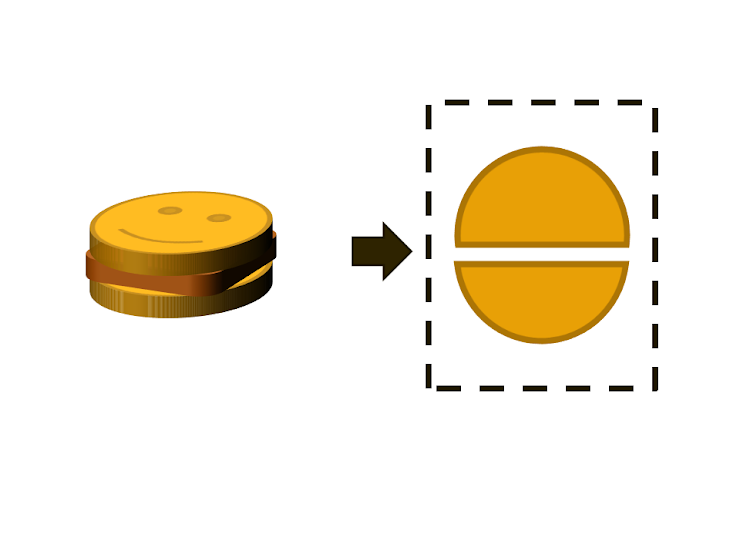How many sandwiches are shown in the box?